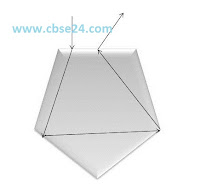# Total Internal Reflection class 10 | cbse24

The light ray are reflect internally. This phenomenon is know as internal reflection, for total internal reflection when light travels from denser to rarer medium and incident angle is more than the critical angle than it completely return this condition is know as total internal reflection.

1;-For first image r>i rays goes from denser to rarer medium and rays refracted

2:- For 2rd image If we increase angle of incident Io at that instant angle of refraction become 90.So Io become Ic(critical angle)

3:-In image 3rd If angle of incident is grater than Ic mean I2>Ic than incident ray bend and angle should more than 90 and back to the same medium and called total internal reflection

Criteria of total internal Reflection:

i=ic,r=900

sin isin r=1μ2=μ2μ1

sinicsin90=μ2μ1

sinic=μ2μ1

where Ic=critical angle

Example:-The high refractive index (2.42) of diamond gives it a critical angle pf only 24 degree ,so may ray trying to cross the diamond air interface are incident at angle greater than the critical angle. The face of diamond are cut in such away that most rays inside the diamond undergo total internal refractionDiamond's total internal reflection

*